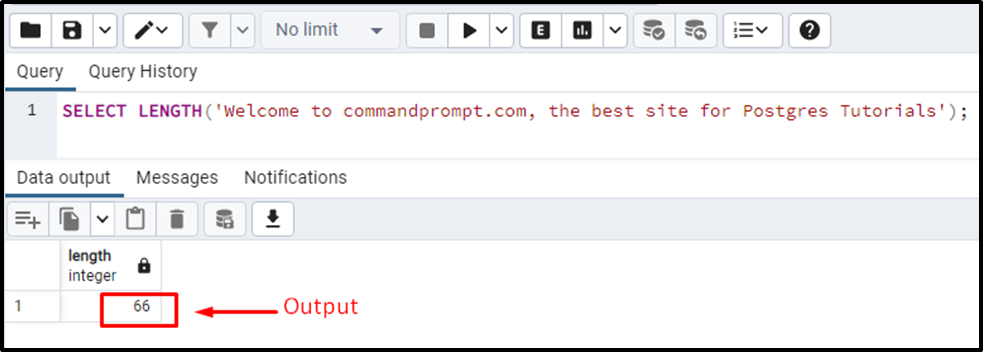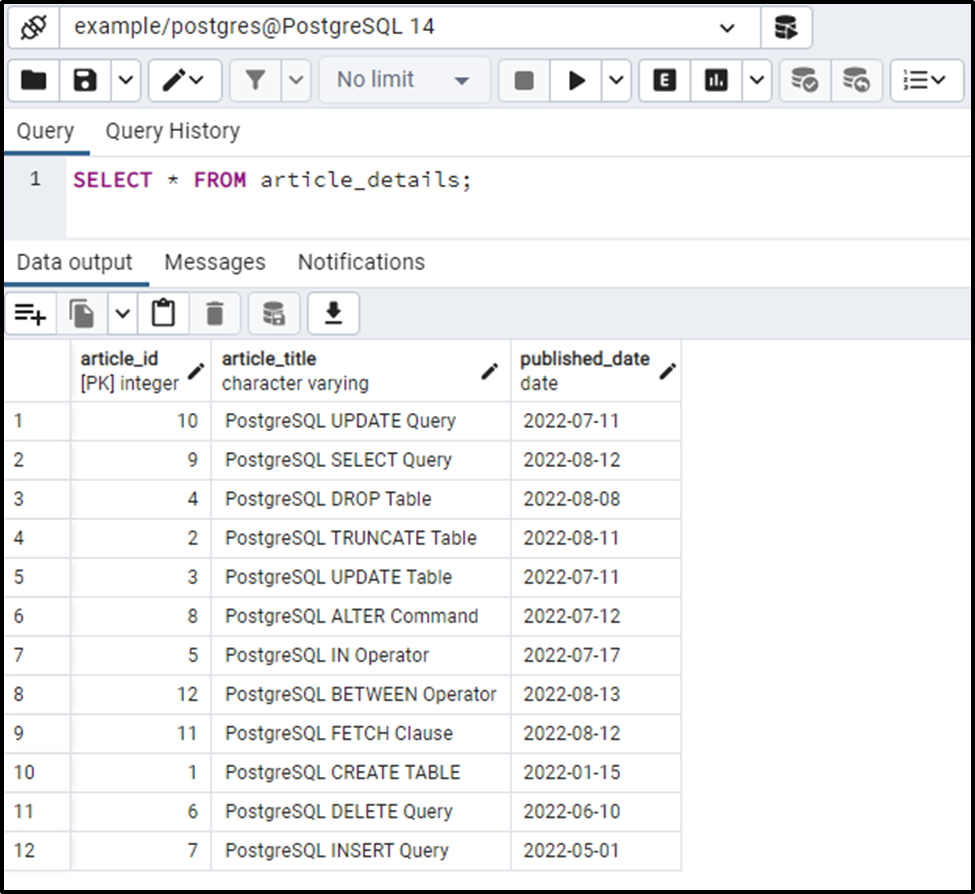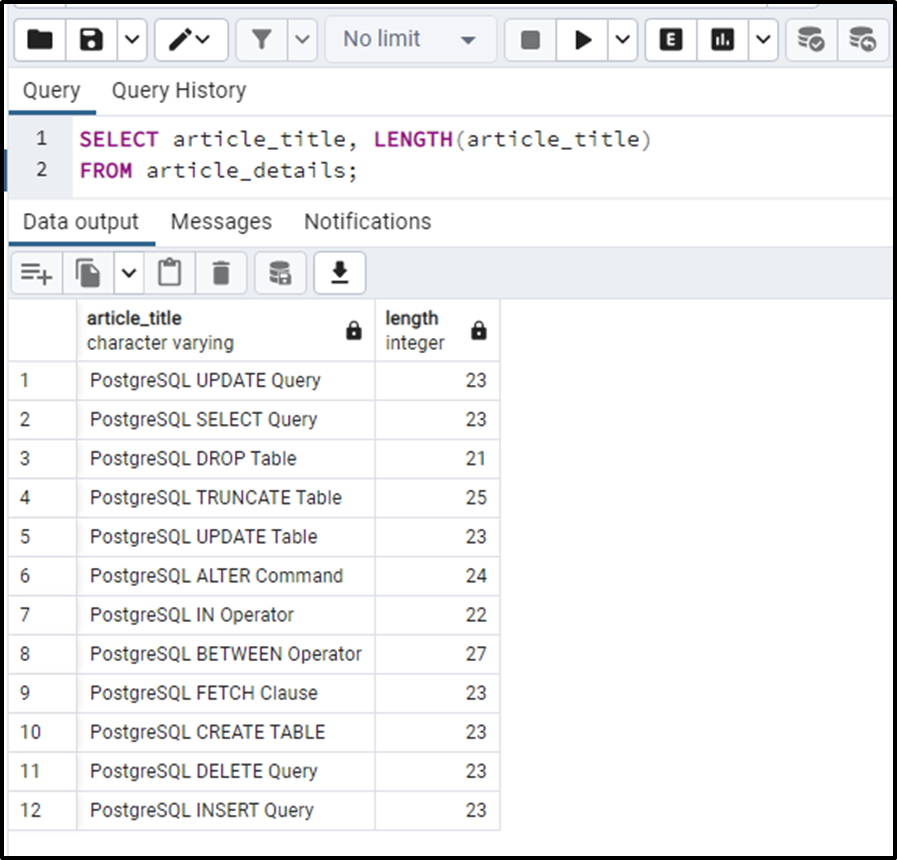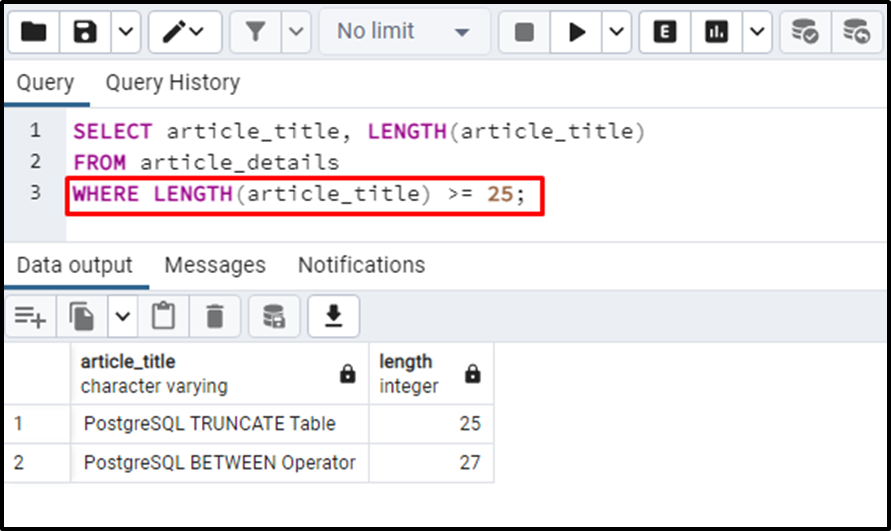##### PostgreSQL LENGTH() Function With Practical Examples

PostgreSQL offers several built-in string functions to calculate the string’s length, for example, the BIT_LENGTH(), CHAR_LENGTH(), etc. Among them, the most popularly used function is the LENGTH() function which takes a string and retrieves its total length. For instance, passing "hello" to the LENGTH() function will return "5", indicating that the input string has five characters.

The purpose of this blog post is to demonstrate how to get the string's length in Postgres using the LENGTH() function. So, let’s get started.

How Does the LENGTH() Function Work in PostgreSQL?

The Postgres LENGTH() function accepts a string as an argument and calculates the total number of characters in that particular string. It retrieves an integer value indicating the total length of the LENGTH() function. In order to use the LENGTH() function in Postgres, users must follow the following syntax:

`LENGTH(‘str’);`

Here, str represents a string whose length needs to be calculated.

Practicing a concept is the best way to understand it. So, let’s do it.

Example 1: How to Use LENGTH() Function in Postgres?

This particular example will show the practical usage of the LENGTH() function:

`SELECT LENGTH('Welcome to commandprompt.com, the best site for Postgres Tutorials');`The output shows that the LENGTH() function retrieves the total length of the input string, including spaces and separators.

Example 2: How to Use LENGTH() Function On Tables Data?

This example is going to teach you how to use the LENGTH() function on a particular table. For this purpose, either you must have an existing table in your database, or you can create a new table. We already have some tables, so we are going to use one of them, i.e., “article_details”:

`SELECT * FROM article_detials;`The selected table has twelve records in it. Let’s utilize the LENGTH() function on the “article_title” column to find the character length of each title:

```SELECT article_title, LENGTH(article_title)
FROM article_details;```The output snippet indicates that the LENGTH() function retrieves the length of each title.

Example 3: How to Use LENGTH() Function With WHERE Clause?

Users can use the LENGTH() function with the collaboration of the WHERE clause to fetch only those strings that meet the length criteria:

```SELECT article_title, LENGTH(article_title)
FROM article_details
WHERE LENGTH(article_title) >= 25;```

The WHERE clause is used in the above query to filter the article titles based on their character length. Only those titles will be fetched whose length is greater than or equal to 25:The output shows that there are only two article titles whose length is greater than or equal to 25.

Conclusion

The Postgres LENGTH() function accepts a string as an argument and calculates the total number of characters in that particular string. It retrieves an integer value indicating the total length of the LENGTH() function. Users can use the LENGTH() function with the collaboration of the WHERE clause to fetch only those strings that meet the length criteria. This blog post demonstrated the working of the LENGTH() function via practical examples.